#### You may also like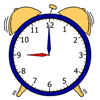### Order, Order!

Can you place these quantities in order from smallest to largest?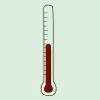### In Order

Can you rank these quantities in order? You may need to find out extra information or perform some experiments to justify your rankings.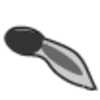### Order the Changes

Can you order pictures of the development of a frog from frogspawn and of a bean seed growing into a plant?

# Escape from the Castle

##### Age 7 to 11 Challenge Level:

Skippy and Anna are locked in a room at the top of a large castle. There are five other locked rooms to go through to get out.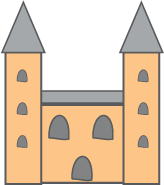The key to that room, and all the other rooms, is a number. The numbers are locked away in a problem. The number is the key to that room.

Can you help them to get out?

You must have the number to be able to do the next problem and you must have all the keys to finish by answering the last question.

#### The first room:

There is a pile of dice.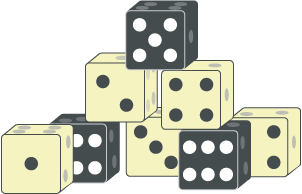Three of them are put in a row. The numbers on the top of these three add to $8$.
What do the hidden numbers on the bottom add to?

This number is the first key.

#### The second room:

Skippy and Anna have the first key number. They have gone through the first locked door.

There are ten cards numbered from $0$ to $9$. Five of these are face down in a row on the table.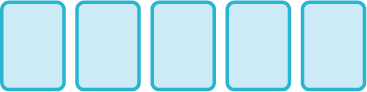The numbers on the first two cards add to the first key number.
The numbers on the second and third cards add to $9$,
the numbers on the third and fourth cards add to $11$,
and the numbers on the fourth and fifth cards add to $16$.

What number is on the last card? This number is the second key.

#### The third room:

Skippy and Anna have the second key number. They have gone through the second locked door. Here there is a diagram.

There are two overlapping circles inside a rectangle. The rectangle is the second key number of centimetres long and $5$ centimetres high.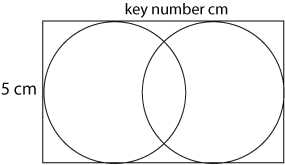How far apart are the centres of the two circles?

Square this answer and subtract one. This will give you the third key number.

#### The fourth room:

Skippy and Anna have the third key number. They have gone through the third locked door.

On the floor there is a strange diagram and the numbers from $1$ to $8$ on eight cards. The diagram is a square with eight boxes arranged round it.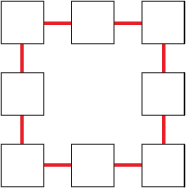Skippy and Anna have to arrange the numbers in the boxes so that each side of the square adds to the third key number.

To find the fourth key number, add the numbers on all the corner boxes and then subtract 10.

#### The fifth room:

Skippy and Anna have the fourth key number. They have gone through the fourth locked door.

On the table there are some jam tarts.

There are also two special trays used to cook them in. These hold the key number of tarts each.

There are enough tarts to fill one tray but not enough to fill the other one as well.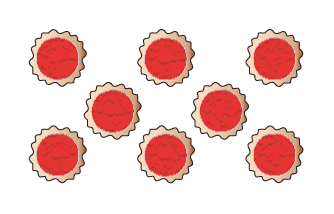If the tarts are counted in fours there are three left over.

If they are counted in threes there is one left over.

How many tarts are there altogether? This number is the fifth and last key.

#### The last question:

However, before they can finally leave the castle you must answer a last question.
What is Skippy's real name?
Using the code $1 = A, 2 = B, 3 = C, ... 26 = Z$ translate all the key numbers into letters.

These letters will give you an anagram of Skippy's real name. When you have worked this out you have finally finished!